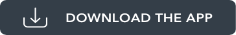You are here: Math API

# Math API

Using the Math API, you can provide your app with the ability to perform various mathematical operations on a given set of numbers.

The Math API uses the `math Namespace` and the following API elements.

Functions

Function Description
Math.max Returns the maximum value among the given set of numbers.
Math.min Returns the minimum value among the given set of numbers.
Math.pow Computes the value of the first parameter raised to the power of the second parameter
Math.sqrt Returns the square root of a given number.
Math.random Generates a real number between 0 and 1.
Math.floor Converts a float value to an integer (number before the decimal).

Properties

Property Description
Math.PI Returns the value of pi.

Find the largest and the smallest numbers from a given set of numbers, by using the Math.max and the Math.min functions. Compute the square root of a number by using the Math.sqrt function and power of a number using the Math.pow function. To return the value of pi, use the Math.pi function. To generate a random number between 0 and 1, use the Math.random function. Further, you can convert a float value to an integer by using the Math.floor function.

To view the functionality of the Math API in action, download the sample application from the link below. Once the application is downloaded, build and preview the application using the Volt MX App.